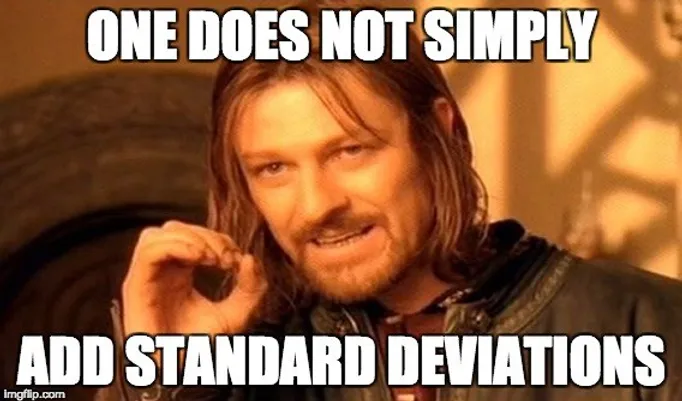top of page

## Chapter 7 - Day 9

##### Learning Targets
• Calculate the mean and standard deviation of the sampling distribution of a difference between sample means.

• If appropriate, use a Normal distribution to calculate probabilities involving a difference between two means.

##### Activity: ACT Scores - Which School is Better?

We like to take the students back to our Skittles vs M&M's lesson to help step the stage for this one. The lesson structure is is very similar to students to try and have them make as many connections as possible. For this lesson, we have found that giving them this reminder at the beginning of class is enough to have them be able to work through the entire activity on their own.

If you wanted to check-in, we would recommend that you have the students stop after question 1 to make sure they have correctly analyzed each of the sampling distributions for AKHS and PHS.

Can't We Just Plug The Numbers Into The Formula?

Sure, we could have started the lesson by giving them the formulas they will need to seeing if they can correctly plug in the numbers. However, we are trying to not make students reliant on the formulas and understand the deeper connections happening. Whenever we work with two distributions, we always want the students to start by thinking about the characteristics of each individual distribution. Using a document like this to help them keep their thoughts organized is a great place to start. That way, they can rely on their knowledge of how we combine random variables to figure out what the new distributions is going to look like.

Teaching Tips

Be prepared for some students to get stuck on question 3 because we haven't given them a "value" to plot on their normal distributions. Ask the students what our difference would be if AKHS and PHS had the same mean score. Ask them what the difference would have to look like for us to know AKHS was higher. Generally, the students catch on that "0" is a dividing point that separates which one scored higher. In this case, we want a positive difference to show AKHS had the higher value.

Students may still want to add standard deviations (or worse subtract them). Continue to drive this fundamental point wherever you can in class:##### Luke's Lesson Notes

Here is a brief video highlighting some key information to help you prepare to teach this lesson.

bottom of page